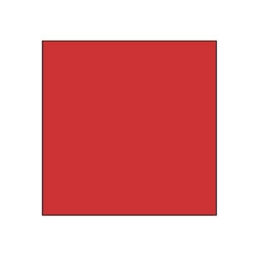# Similarity 30821

There is a given square ABCD with a = 5.3cm. Determine the side size of a similar square if the similarity ratio k = 3cm. Calculate the area and the perimeter of the magnified square

S =  252.81 cm2
o =  63.6 cm

### Step-by-step explanation:Did you find an error or inaccuracy? Feel free to write us. Thank you!

#### You need to know the following knowledge to solve this word math problem:

We encourage you to watch this tutorial video on this math problem: